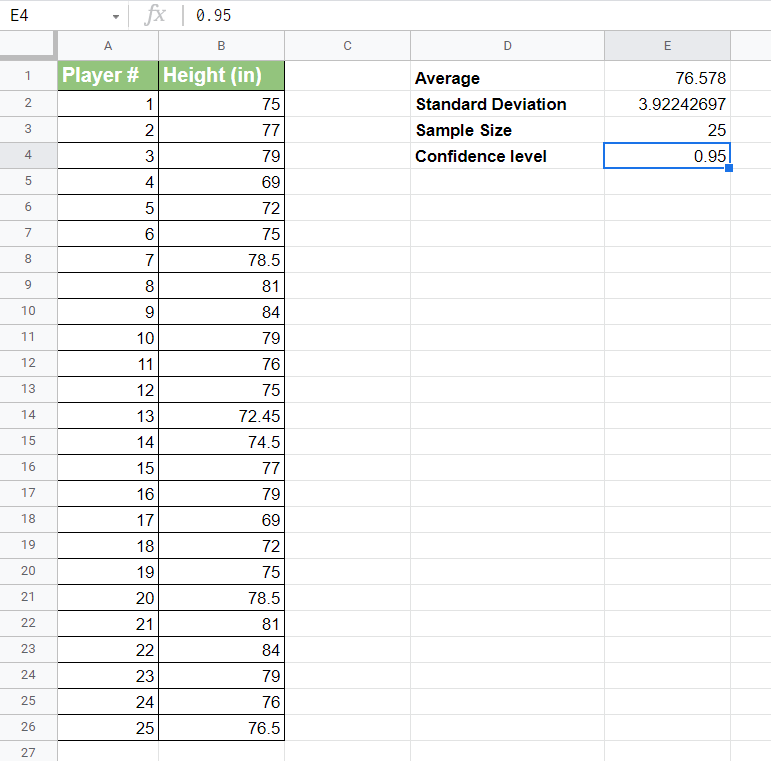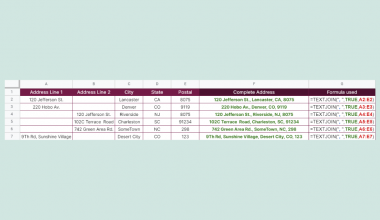How to Calculate the Confidence Interval in Google Sheets

Knowing how to calculate the confidence interval in Google Sheets is useful when you want to measure the degree of uncertainty in your sampling method.

The confidence interval is often used by statisticians to understand whether a sample dataset can be trusted as a true representation of the population.

But first, what exactly is a confidence interval?

The confidence interval refers to how far the sample mean is from the actual population mean. It essentially displays the error interval between these two means. This means that the interval is within a specific upper and lower error limit.

If you are calculating for a 90% confidence interval, this means that you can be 90% certain that the population mean lies in the sample mean interval. Usually, 95% and 99% confidence intervals are used to minimize the error percentage.

Let’s take a quick example to further illustrate this concept.

Let’s say you are a researcher looking into the heights of NBA players. You first take a random sample from the population and calculate a mean height of 80in.

This mean height does not really give an indication of the population mean. The average mean of the sample heights is simply a point estimate which doesn’t tell you how much it deviates from the population mean.

However, if we establish a confidence level of 95% and a normal distribution (bell-curve), we can arrive at an upper and lower bound which we can guarantee to contain the true mean 95% of the time.

Let’s say that after our computation, we’ve arrived at an interval between 74 and 86 inches. This means that if we were to take 100 random samples from the populations, the mean should fall between 76in and 84in, in all but 5 of those examples.

If we decide to go for a 99% confidence interval, then that means that after 100 random samples, we should get a mean within the interval 99% of the time.

In order to calculate the interval in Google Sheets, we’ll need several statistical functions, including TINV, AVERAGE, STDEV, and COUNT. We’ll be using these functions to replicate the mathematical formula for confidence interval, as seen below:Let’s learn how to solve for the confidence interval ourselves in Google Sheets. Later, we’ll test out our formula using an actual dataset.

Calculating the Confidence Interval in Google Sheets

Let’s look at a real example of a Google Sheets spreadsheet used to calculate the confidence interval.

In the spreadsheet below, we have a sample of 25 players and their heights. We could chart a histogram of the data, but we still have a vague idea of how confident we can be with our sample mean. Using the confidence interval formula, we were able to get the lower and upper bounds of a 95% confidence interval.To get the lower bound, we just need to use the following formula:

= E1 - TINV(1-E4, E3-1)*E2/SQRT(E3)

And to get the upper bound, we use the following:

=E1 + TINV(1-E4, E3-1)*E2/SQRT(E3)

In this second example, we adjust the parameters so that we can find the upper and lower bound of a 99% confidence interval.

If you’re ready to solve for the confidence interval in Google Sheets, check out the next section for a step-by-step guide.

How to Calculate the Confidence Interval in Google Sheets

In this section, we will go through each step needed to start solving the confidence interval. This guide will use the same height dataset used in the earlier examples.

Follow these five simple steps to get the confidence interval:

1. First, let’s find the mean of the sample using the AVERAGE function. In this example, the average height of the sample is 76.578 in.2. Next, we’ll need to get the standard deviation of the sample. We can use the STDEV function to quickly solve this.3. In the next step, we use the COUNT function to get the sample size. In this example, we have a sample size of 25 players.4. Next, we’ll have to indicate the confidence level we want to use to calculate our interval. In this example, let’s try solving for a 95% confidence interval.5. Finally, we can use the confidence interval formula to get the upper and lower bound.Our computation tells us that the interval lies between 74.95 in and 78.197 in.

1. What is the difference between confidence interval and confidence level?
These two concepts are similar, but there is a slight difference. The confidence level refers to the percentage you expect to get close to the same estimate if you run a certain experiment or sampling. For example, with a confidence level of 99%, you expect the result to be the same 99 times out of 100.
The confidence interval refers to the upper and lower bounds of the estimate you expect to find at a particular level of confidence. The interval gives us a range of values we might expect at a certain confidence level. If we have an interval of 15 to 20 at a 95% interval, that means that the value returned will be within that range 95 times out of 100.

Now you know how to easily calculate the confidence interval in Google Sheets. The confidence interval values can help determine the range to expect if you were to take a sample again.

The confidence interval formula is just one example of a statistical use case for function in Google Sheets. With so many other Google Sheets functions out there, you can surely find one that suits your use case.

Are you interested in learning more about what Google Sheets can do? Make sure to subscribe to our newsletter to be the first to know about the latest guides and tutorials from us.Our goal this year is to create lots of rich, bite-sized tutorials for Google Sheets users like you. If you liked this one, you'd love what we are working on! Readers receive ✨ early access ✨ to new content.

You May Also LikeHow to Use AVERAGE.WEIGHTED Function in Google Sheets

The AVERAGE.WEIGHTED function in Google Sheets is useful when trying to find the average of a data set…How to Use DAVERAGE Formula in Google Sheets

The DAVERAGE formula in Google Sheets is useful to get the average of values that meet specific criteria.…How to Count Cells Based on the Cell Color in Google Sheets

While there are no built-in functions in Google sheets to count cells based on the cell color, you…

How To Use ISNONTEXT Function in Google Sheets

The ISNONTEXT function in Google Sheets is used when you need to determine whether the value of a…